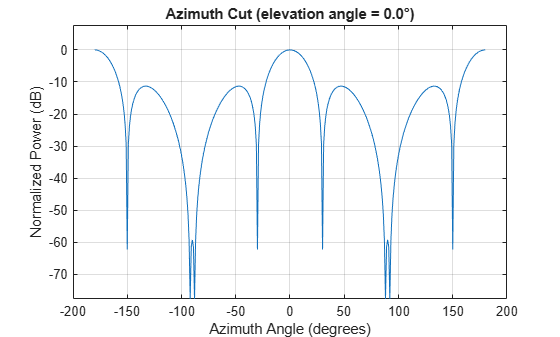# phased.ArrayResponse

Sensor array response

## Description

The `ArrayResponse` object calculates the complex-valued response of a sensor array.

To compute the response of the array for specified directions:

1. Define and set up your array response calculator. See Construction.

2. Call `step` to estimate the response according to the properties of `phased.ArrayResponse`. The behavior of `step` is specific to each object in the toolbox.

Note

Starting in R2016b, instead of using the `step` method to perform the operation defined by the System object™, you can call the object with arguments, as if it were a function. For example, ```y = step(obj,x)``` and `y = obj(x)` perform equivalent operations.

## Construction

`H = phased.ArrayResponse` creates an array response System object, `H`. This object calculates the response of a sensor array for the specified directions. By default, a 2-element uniform linear array (ULA) is used.

`H = phased.ArrayResponse(Name,Value)` creates object, `H`, with each specified property Name set to the specified Value. You can specify additional name-value pair arguments in any order as (`Name1`,`Value1`,...,`NameN`,`ValueN`).

## Properties

 `SensorArray` Handle to sensor array used to calculate response Specify the sensor array as a handle. The sensor array must be an array object in the `phased` package. The array can contain subarrays. Default: `phased.ULA` with default property values `PropagationSpeed` Signal propagation speed Specify the propagation speed of the signal, in meters per second, as a positive scalar. Default: Speed of light `WeightsInputPort` Add input to specify weights To specify weights, set this property to `true` and use the corresponding input argument when you invoke `step`. If you do not want to specify weights, set this property to `false`. Default: `false` `EnablePolarization` Enable polarization simulation Set this property to `true` to let the array response simulate polarization. Set this property to `false` to ignore polarization. This property applies only when the array specified in the `SensorArray` property is capable of simulating polarization. Default: `false`

## Methods

 step Calculate array response of sensor array
Common to All System Objects
`release`

Allow System object property value changes

## Examples

collapse all

Calculate array response for a 4-element uniform linear array (ULA) in the direction of 30 degrees azimuth and 20 degrees elevation. Assume the array's operating frequency is 300 MHz.

Construct ULA and ArrayResponse System objects

```fc = 300e6; c = physconst('LightSpeed'); array = phased.ULA(4); response = phased.ArrayResponse('SensorArray',array); resp = response(fc,[30;20])```
```resp = 0.2768 ```

Plot the array response in dB

Plot the normalized power in db as an azimuth cut at 0 degrees elevation.

`pattern(array,fc,[-180:180],0,'PropagationSpeed',c,'CoordinateSystem','rectangular','Type','powerdb')`## References

 Van Trees, H. Optimum Array Processing. New York: Wiley-Interscience, 2002.